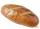On the farm

On the farm were housed 70 heads of cattle with food supply for 180 days. After 60 days, 10 head of cattle sold, and for the next 30 days they sold 10 more. How many days is sufficient supply of feed for the remaining cattle?

Result

x =  132 d

Solution:Leave us a comment of this math problem and its solution (i.e. if it is still somewhat unclear...):Be the first to comment!To solve this verbal math problem are needed these knowledge from mathematics:

Do you have a linear equation or system of equations and looking for its solution? Or do you have quadratic equation?

Next similar math problems:

1. Bed timeTiffany was 5 years old; her week night bedtime grew by ¼ hour each year. If, at age 18, her curfew time is 11pm, what was her bed time when she was 5 years old?
2. Find xSolve: if 2(x-1)=14, then x= (solve an equation with one unknown)
3. Simple equationSolve the following simple equation: 2. (4x + 3) = 2-5. (1-x)
4. Simple equationSolve for x: 3(x + 2) = x - 18
5. Dropped sheetsThree consecutive sheets dropped from the book. The sum of the numbers on the pages of the dropped sheets is 273. What number has the last page of the dropped sheets?
6. AlleyAlley measured a meters. At the beginning and end are planted poplar. How many we must plant poplars to get the distance between the poplars 15 meters?
7. HotelThe hotel has a p floors each floor has i rooms from which the third are single and the others are double. Represents the number of beds in hotel.
8. Forest nurseryIn the forest nursery after winter, they found that 1/10 stems died out of them. For them, they land 193 new spruces. How many spruces are in the forest nursery?
9. SimplifySimplify the following problem and express as a decimal: 5.68-[5-(2.69+5.65-3.89) /0.5]
10. Hectoliters of waterThe pool has a total of 126 hectoliters of water. The first pump draws 2.1 liters of water per second. A second pump pumps 3.5 liters of water per second. How long will it take both pumps to drain four-fifths of the water at the same time?5 of the same bread has the same weight as three bread and 4 kg of fruit. What weight has one bread?Solve for a specified variable: P=a+4b+3c, for aSolve the following equation: 36=-(1+7x)-6(-7-x)The student who asked how many years he answered: "After 10 years I will be twice as old than as I was four years ago. How old is student?That number increased by three equals three times itself?Given 2x =0.125 find the value of xSolve next equation: 2 ( 2x + 3 ) = 8 ( 1 - x) -5 ( x -2 )